# SSC CGL 2018 Practice Test Papers | Quantitative Aptitude (Day-1)

Dear Aspirants, Here we have given the Important SSC CGL Exam 2018 Practice Test Papers. Candidates those who are preparing for SSC CGL 2018 can practice these questions to get more confidence to Crack SSC CGL 2018 Examination.

[WpProQuiz 2596]

Click “Start Quiz” to attend these Questions and view Explanation

1. The ratio of speed of boat and speed of current is 5:1. The boat takes 80 minutes to go from one end of the river and return back. What is the speed of boat if the time taken for downstream is 32 minutes and the distance between one end and the other end of the river is 3.2 km.
1. 4 km/hr
2. 2 km/hr
3. 3 km/hr
4. 5 km/hr
1. An angle is 3 times its supplementary angle. What is the measure of that angle?
1. 135
2. 125
3. 150
4. 120
1. The ratio of acid and mixture of acid and distilled water is 1:5. How many liters of distilled water to be replaced to make the concentration of acid to 30% if initially there was 32 liters of distilled water?
1. 4
2. 8
3. 12
4. 15
1. Ankit, Ajay and Anil have factories in the ratio 2:5:3. Due to loss in business they sold some of their factories in the ratio 1:4:5 respectively. What is the number of factories Ajay have now if they have initially 200 factories in total and 170 factories now?
1. 88
2. 56
3. 78
4. 48
1. In a coffee shop there is a offer on valentine’s day i.e. 15% discount on total rate for couple. Mr. & Mrs. Raj ordered cold coffee which costs 200 each and Mr. & Mrs. Harish ordered Espresso which costs 150 each. What is the bill amount for the both couples’ order?
1. 515
2. 297.5
3. 395
4. 595
1. The average of 10, 20, 30, 40 ….100 is 55. If each number is multiplied by 2 and then subtracted by 5 what is the new average?
1. 95
2. 100
3. 105
4. 110
1. The average age of 20 English professors in a college is 44 years, the number of Maths Professors is three fourth of the number of English professors then what is the average age of Maths professors?
1. 88
2. 33
3. None of these
4. Cannot be determined
1. Brinda travels from Gurgaon to Agartala at the speed of 40 km/hr and returns from Agartala to Gurgaon at the speed of x km/hr. Find the average speed of Brinda if the ratio of sum of two speeds i. e. to and from and difference of two speeds is 5:2. (x>40)
1. 48 km/hr
2. 50 km/hr
3. 40 km/hr
4. 32 km/hr
1. The cost of 3 BHK flat is three fourth of difference between 1 BHK flat and a villa. The cost of 1 BHK flat and villa is 40 lakh and 1.4 crore respectively and the cost of 2 BHK flat is two third of the cost of 3 BHK flat. What is the sum of the costs of 2 BHK flat and 1 BHK flat?
1. 80 lakh
2. 75 lakh
3. 90 lakh
4. 1 crore
1. 5 years ago the average age of family of 5 members is 32 years. Krish got married today and the average increases by 3. What is the age of Mrs. Krish?
1. 35
2. 30
3. 25
4. Cannot be determined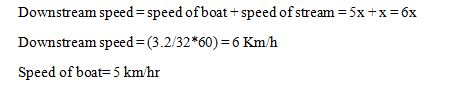x+3x=180

x=45

3x=135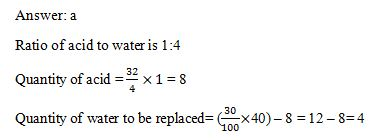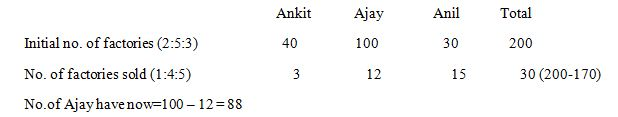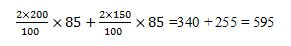55*2-5=105

There is no Average Weight of Maths professors’ given.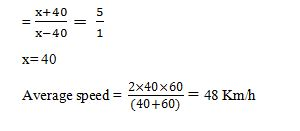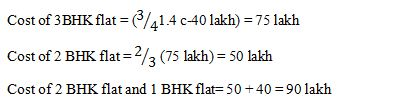Present average of five members = 32+5=37

Sum of ages of five members = 37*5 = 185

Age of Mrs. Krish => (185+x) /6 = 25

Age of Mrs. Krish = 25